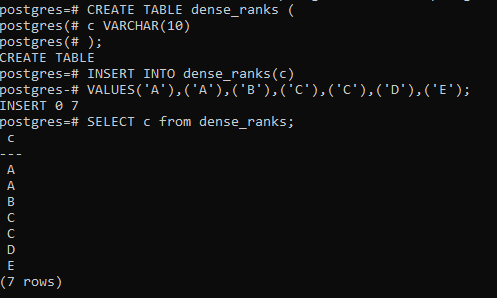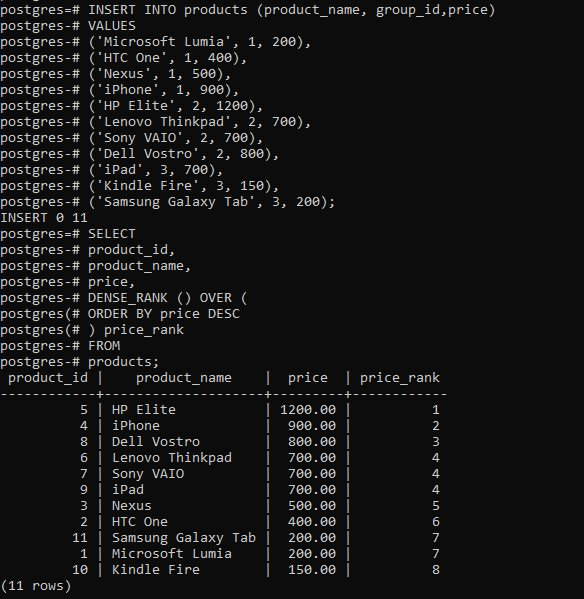Related Articles

# PostgreSQL- DENSE_RANK Function

• Last Updated : 28 Aug, 2020

In PostgreSQL, the DENSE_RANK() function is used to assign a rank to each row within a partition of a result set, with no gaps in ranking values. The DENSE_RANK() assigns a rank to every row in each partition of a result set. Different from the RANK() function, the DENSE_RANK() function always returns consecutive rank values. For each partition, the DENSE_RANK() function returns the same rank for the rows which have the same values

The following shows the syntax of the DENSE_RANK() function:

```Syntax:
DENSE_RANK() OVER (
[PARTITION BY partition_expression, ... ]
ORDER BY sort_expression [ASC | DESC], ...
)
```

The DENSE_RANK() function is applied to every row in each partition defined by the PARTITION BY clause, in the sort order specified by the ORDER BY clause. It will reset the rank when crossing the partition boundary. The PARTITION BY clause is optional. If you skip it, the DENSE_RANK() function will treat the whole result set as a single partition.

Example 1:

First, create a table named dense_ranks that has one column:

```CREATE TABLE dense_ranks (
c VARCHAR(10)
);
```

Now insert some data into it:

```INSERT INTO dense_ranks(c)
VALUES('A'), ('A'), ('B'), ('C'), ('C'), ('D'), ('E');
```

Now query from the dense_ranks table:

```SELECT c from dense_ranks;
```

It will result in the below depiction:Fourth, use the DENSE_RANK() function to assign a rank to each row in the result set:

```SELECT
c,
DENSE_RANK() OVER (
ORDER BY c
) dense_rank_number
FROM
dense_ranks;
```

Output:Example 2:

First, create two tables named products and product_groups for the demonstration:

```CREATE TABLE product_groups (
group_id serial PRIMARY KEY,
group_name VARCHAR (255) NOT NULL
);

CREATE TABLE products (
product_id serial PRIMARY KEY,
product_name VARCHAR (255) NOT NULL,
price DECIMAL (11, 2),
group_id INT NOT NULL,
FOREIGN KEY (group_id) REFERENCES product_groups (group_id)
);
```

Now insert some data to the table:

```INSERT INTO product_groups (group_name)
VALUES
('Smartphone'),
('Laptop'),
('Tablet');

INSERT INTO products (product_name, group_id, price)
VALUES
('Microsoft Lumia', 1, 200),
('HTC One', 1, 400),
('Nexus', 1, 500),
('iPhone', 1, 900),
('HP Elite', 2, 1200),
('Sony VAIO', 2, 700),
('Dell Vostro', 2, 800),
('Kindle Fire', 3, 150),
('Samsung Galaxy Tab', 3, 200);
```

The below statement uses the DENSE_RANK() function to rank products by list prices:

```SELECT
product_id,
product_name,
price,
DENSE_RANK () OVER (
ORDER BY price DESC
) price_rank
FROM
products;
```

Output:My Personal Notes arrow_drop_up